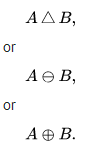﻿ Python: Create a symmetric difference - w3resource# Python: Create a symmetric difference

## Python sets: Exercise-9 with Solution

Write a Python program to create a symmetric difference.

From Wikipedia,
In mathematics, the symmetric difference, also known as the disjunctive union, of two sets is the set of elements which are in either of the sets and not in their intersection. The symmetric difference of the sets A and B is commonly denoted byFor example, the symmetric difference of the sets {1,2,3} and {3,4} is {1,2,4}.Sample Solution-1:

Using symmetric_difference() Method

Python Code:

``````setc1 = set(["green", "blue"])
setc2 = set(["blue", "yellow"])
print("Original sets:")
print(setc1)
print(setc2)
r1 = setc1.symmetric_difference(setc2)
print("\nSymmetric difference of setc1 - setc2:")
print(r1)
r2 = setc2.symmetric_difference(setc1)
print("\nSymmetric difference of setc2 - setc1:")
print(r2)
setn1 = set([1, 1, 2, 3, 4, 5])
setn2 = set([1, 5, 6, 7, 8, 9])
print("\nOriginal sets:")
print(setn1)
print(setn2)
r1 = setn1.symmetric_difference(setn2)
print("\nSymmetric difference of setn1 - setn2:")
print(r1)
r2 = setn2.symmetric_difference(setn1)
print("\nSymmetric difference of setn2 - setn1:")
print(r2)
```
```

Sample Output:

```Original sets:
{'blue', 'green'}
{'blue', 'yellow'}

Symmetric difference of setc1 - setc2:
{'yellow', 'green'}

Symmetric difference of setc2 - setc1:
{'yellow', 'green'}

Original sets:
{1, 2, 3, 4, 5}
{1, 5, 6, 7, 8, 9}

Symmetric difference of setn1 - setn2:
{2, 3, 4, 6, 7, 8, 9}

Symmetric difference of setn2 - setn1:
{2, 3, 4, 6, 7, 8, 9}
```

Pictorial Presentation:## Visualize Python code execution:

The following tool visualize what the computer is doing step-by-step as it executes the said program:

Sample Solution-2:

Using symmetric difference operator(^)

Python Code:

``````setc1 = set(["green", "blue"])
setc2 = set(["blue", "yellow"])
print("Original sets:")
print(setc1)
print(setc2)
r1 = setc1^setc2
print("\nSymmetric difference of setc1 - setc2:")
print(r1)
r2 = setc2^setc1
print("\nSymmetric difference of setc2 - setc1:")
print(r2)
setn1 = set([1, 1, 2, 3, 4, 5])
setn2 = set([1, 5, 6, 7, 8, 9])
print("\nOriginal sets:")
print(setn1)
print(setn2)
r1 = setn1^setn2
print("\nSymmetric difference of setn1 - setn2:")
print(r1)
r2 = setn2^setn1
print("\nSymmetric difference of setn2 - setn1:")
print(r2)
```
```

Sample Output:

```Original sets:
{'blue', 'green'}
{'yellow', 'blue'}

Symmetric difference of setc1 - setc2:
{'yellow', 'green'}

Symmetric difference of setc2 - setc1:
{'yellow', 'green'}

Original sets:
{1, 2, 3, 4, 5}
{1, 5, 6, 7, 8, 9}

Symmetric difference of setn1 - setn2:
{2, 3, 4, 6, 7, 8, 9}

Symmetric difference of setn2 - setn1:
{2, 3, 4, 6, 7, 8, 9}
```

Pictorial Presentation:## Visualize Python code execution:

The following tool visualize what the computer is doing step-by-step as it executes the said program:

Python Code Editor:

Have another way to solve this solution? Contribute your code (and comments) through Disqus.

What is the difficulty level of this exercise?

Test your Programming skills with w3resource's quiz.

﻿

## Python: Tips of the Day

Debug With the Print() Function:

```>>> for i in range(5):
...     print(i, end=', ' if i < 4 else '\n')
...
0, 1, 2, 3, 4
>>> for i in range(5):
...     print(f'{i} & {i*i}', end=', ' if i < 4 else '\n')
...
0 & 0, 1 & 1, 2 & 4, 3 & 9, 4 & 16
```